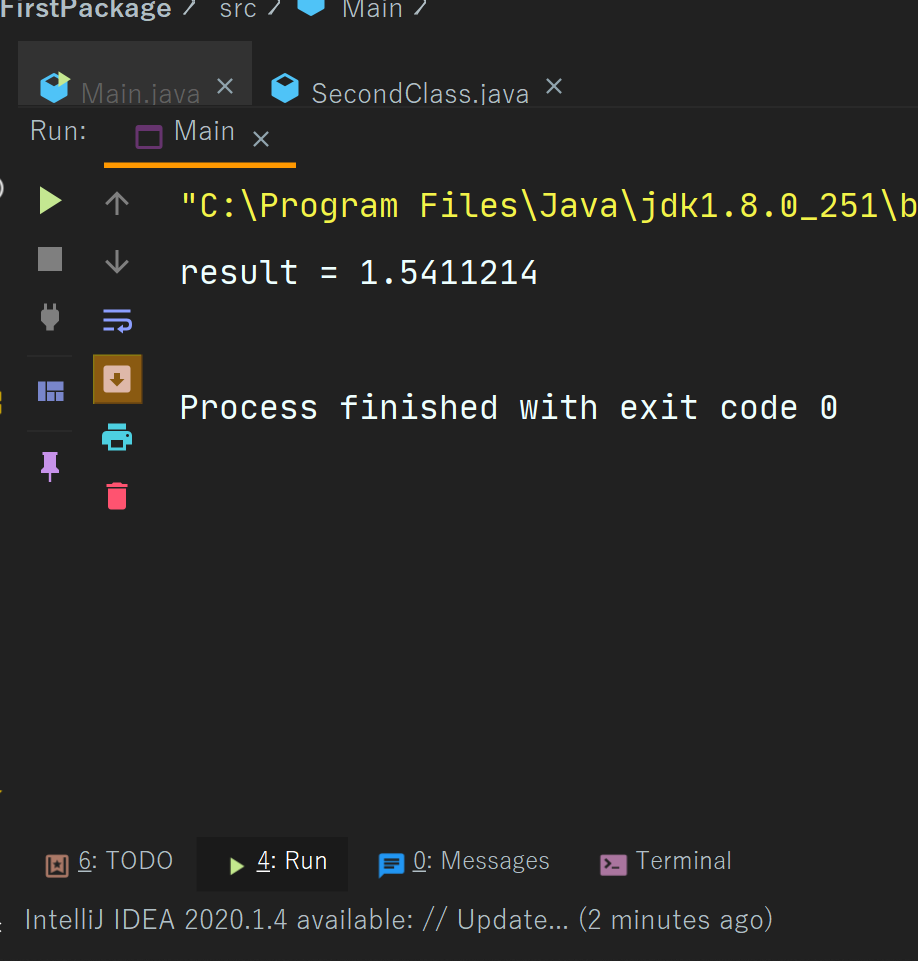Java Division Operators

# Java Division Operators

Division Operator In Java
The division operator in java is represented by the forward-slash sign which is indicated as  - “ / ”.
The division operator is used to divide a value from another value.
It is also a part of the binary arithmetic operator which means that this operator is used on two operators.
Now we will see Example -
Example -
 import second.*; import java.util.*; // division operator here class Main{   public static void main(String[] args) {       float a = 34.23f;       float b = 22.2111f;       float result  = a/b;       System.out.println("result = "+result);       }   }
Output -